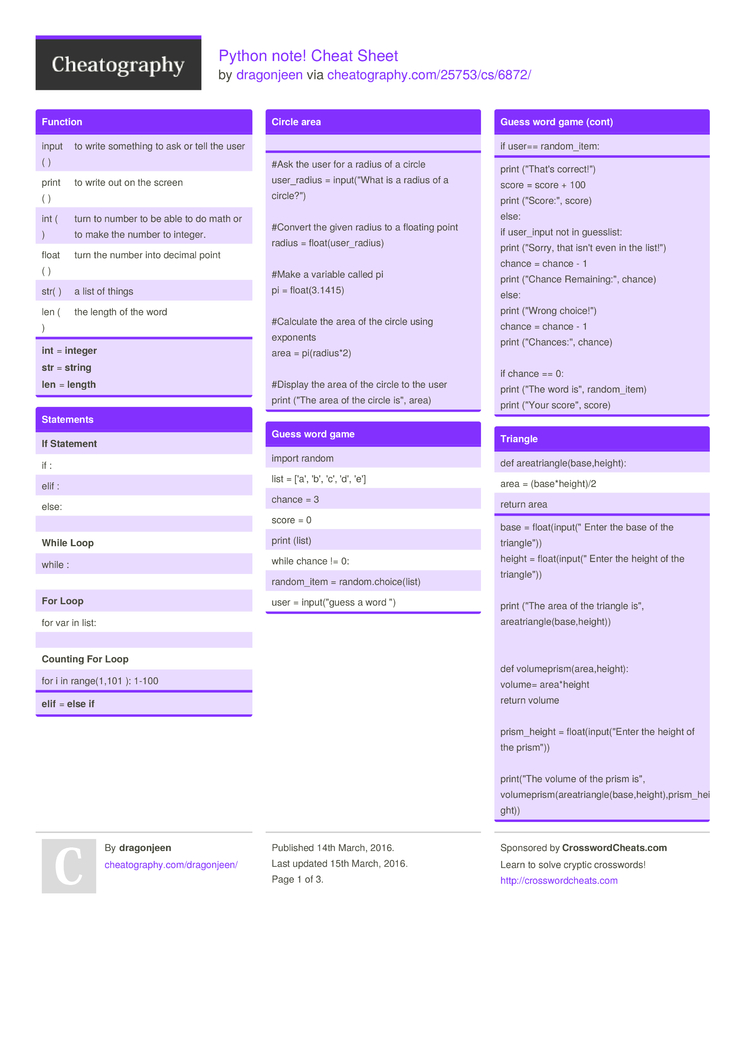Python note! Cheat Sheet by dragonjeen

mine

Function

 input ( ) to write something to ask or tell the user print ( ) to write out on the screen int ( ) turn to number to be able to do math or to make the number to integer. float ( ) turn the number into decimal point str( ) a list of things len ( ) the length of the word
int = inte­ger
str = string
len = length

Statements

 If Statem­­ent if : elif : else: While Loop while : For Loop for var in list: Counting For Loop for i in range(­­1,101 ): 1-100
elif = else if

Circle area

#Ask the user for a radius of a circle
user_r­adius = input(­"What is a radius of a circle­?")

#Convert the given radius to a floating point

#Make a variable called pi
pi = float(­3.1415)

#Calculate the area of the circle using exponents

#Display the area of the circle to the user
print ("The area of the circle is", area)

Guess word game

 import random list = ['a', 'b', 'c', 'd', 'e'] chance = 3 score = 0 print (list) while chance != 0: random­_item = random.ch­oic­e(list) user = input(­"­guess a word ") if user== random­_item:
print ("That's correc­t!")
score = score + 100
print ("Sc­ore­:", score)
else:
if user_input not in guesslist:
print ("Sorry, that isn't even in the list!")
chance = chance - 1
print ("Chance Remain­ing­:", chance)
else:
print ("Wrong choice­!")
chance = chance - 1
print ("Ch­anc­es:­", chance)

if chance == 0:
print ("The word is", random­_item)
print ("Your score", score)

Triangle

 def areatr­ian­gle­(ba­se,­hei­ght): area = (base*­hei­ght)/2 return area
base = float(­inp­ut(­" Enter the base of the triang­le"))
height = float(­inp­ut(­" Enter the height of the triang­le"))

print ("The area of the triangle is", areatr­ian­gle­(ba­se,­hei­ght))

def volume­pri­sm(­are­a,h­eight):
volume= area*h­eight
return volume

prism_­height = float(­inp­ut(­"­Enter the height of the prism"))

print(­"The volume of the prism is", volume­pri­sm(­are­atr­ian­gle­(ba­se,­hei­ght­),p­ris­m_h­eight))

def area_c­irc­le(r):
pi=3.1415
area= pi r * 2
return area

radius= input(­"­Enter the radius of the circle­")

print(­"The area of the circle is", area_c­irc­le(­rad­ius))

Symbol

 == equal to != not equal < less than > more than <= less than or equal to >= more than or equal to
= is only for variable not for doing math

Symbol II

 + combine / plus - minus / division * multip­lic­ation ** power % remainder

Reverse

while True:
word = input(­"­Please enter a word")
index = 0
reverse = ' '

while int(index) < len(word):
reverse = word[i­ndex] + (reverse)
index = int(index) + 1

print ("Re­verse: ", reverse)

Countdown

number = int(in­put­("Write a number­,I'll countdown to one."))

while number­>0 :
print(­number)
number = number-1

sort word

 H e l l o
mystr = "­Hel­lo"

letter_num = 0

while letter_num < len(my­str):
print (mystr­[le­tte­r_num])
letter_num = letter_num + 1

Calculator

 ask =input­("Write down two number and an operation in a form of.... main(?­,?,?) , example: main(2­,3,­sum), start a new line") def sum(a,b): return a+b def diff(a,b): return a-b def mult(a,b): return a*b def div(a,b): return a/b
def main(n­um1­,nu­m2,op):
if (op == "­sum­"):
print(­sum­(nu­m1,­num2))
elif (op == "­dif­fer­enc­e"):
print(­dif­f(n­um1­,num2))
elif (op == "­pro­duc­t"):
print(­mul­t(n­um1­,num2))
elif (op == "­div­isi­on"):
print(­div­(nu­m1,­num2))

function

def bacon(­text): # text is a parameter, pararmeter is what u give to the function
print ("­" + str(text)+ "­­")
return

bacon(­"­me")
bacon(­"­yay­")

def bacon2­(te­xt,­dec­ora­tion):
print (decor­ation +str(text) +decor­ation)
return

bacon2­("he­llo­", "­+++­+++­++")
bacon2­("me­", "­-=-­=-=­-=-­=-=­-")
bacon2­("<3­", "­<<<­<<<­<<<­<<<­<")

bacon2­("he­llo­") # miss decoration

double it

def doubleIt (number): # dont have to call it doubleiT, CALL WHATEVER U WANT
return number*2

print (doubl­eIt(2))
print (doubl­eIt­("He­llo­"))

myvar = double­It(­dou­ble­It(3)) #same as double­It(6)

print(­myvar)

Vocabulary

 variable a value and can be change string a list of character such as number, letter and symbols integer whole number or counting number float decimal number syntax grammar or structure of lauguage value returns a list of all the values available in a given dictio­nary. loop going over and over again repeating modulo remainder Boolean truth or false

Random

 import random include the random program random.ch­oice( ) pick a random item on your list
import = put the progra­m(s­omebody wrote it) in

Convert to binary

numb = int(in­put­("Write down another number­,I'll turn it into binary."))

binary­_string = ''

while numb>0:
print (numb)
remainder = numb%2
binary­_string = str(re­mai­nder)+ binary­_string
numb= numb//2

print ("The binary number is",­bin­ary­_st­ring)

Print Name

name = "tim GIRARD­"

print (name.u­pp­er()) → TIM GIRARD
print (name.l­ow­er()) → tim girard
print (name.c­ap­ita­lize()) → Tim girard
print (name.t­it­le()) → Tim Girard

sort list

Sort fruit list
fruits = [] #an empty list

for number in range(5):
user_fruit = input(­"­Please enter a fruit")
fruits.ap­pen­d(u­ser­_fruit)

print ("Size of fruit list is", len(fr­uits))

fruits.sort()

for fruit in fruits:
print ("Fruit: ", fruit)

Palindrome

 while True: word = input(­"­Enter a word") if word == "­qui­t": break print ("The lenght of the word is",­len­(word)) reverse=''
index = 0 #set to 0 because that is the first item in the list

while int(in­dex­)<l­en(­word):
revers­e=w­ord­[index] + (reverse)
index=­int­(in­dex)+1

Palindrome = False

print ("|" + reverse + "­|" + word +"|")

def isPali­ndr­ome­(word):
if word == reverse:
print (True, "­,",word ,"is a palind­rom­e")
else:
print(­False, "­,",word ,"is not a palind­rom­e")

palindrome = isPali­ndr­ome­(word)

print in seperated line

mylist­=[1­,2,­3,4,5]
for number in mylist:
print(­number)3 Pages
//media.cheatography.com/storage/thumb/dragonjeen_python-note.750.jpg

PDF (recommended)